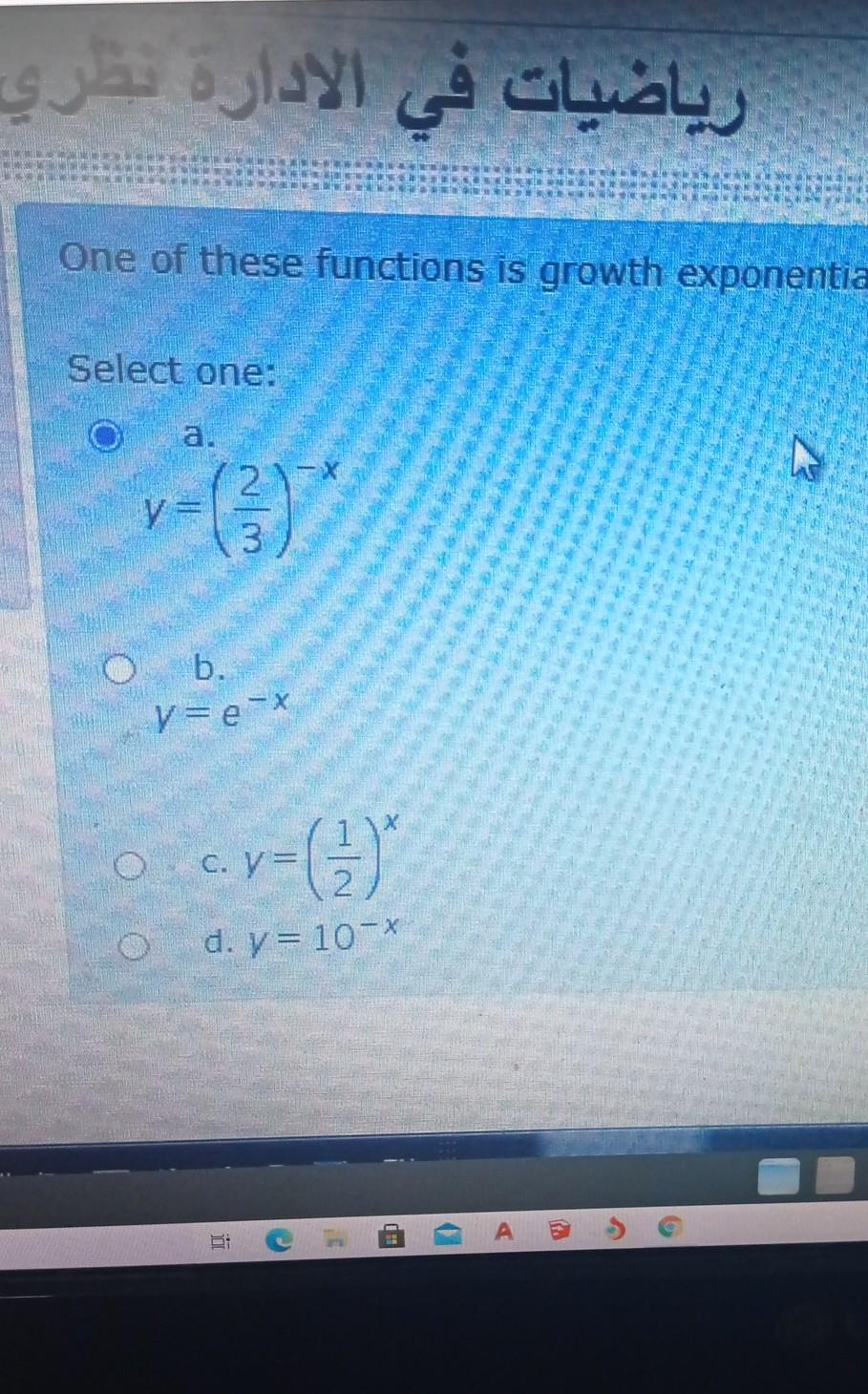### Create an Account

Home / Questions / رياضيات في الادارة سري One of these functions is growth exponentia Select one: WIN b. v=e-...

# رياضيات في الادارة سري One of these functions is growth exponentia Select one: WIN b. v=e-* C, V- 7= (3) d. = 10-X - م

رياضيات في الادارة سري One of these functions is growth exponentia Select one: WIN b. v=e-* C, V- 7= (3) d. = 10-X - مMay 28 2021 View more View LessSubscribe To Get Solution